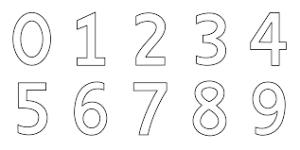# How many 6 digits can be formed puzzle

664.2K Views

How many six digit numbers can be formed using the digits 1 to 6, without repetition such that the number is divisible by the digit at its unit place?Explanation-
XXXXX1 is always divisible by 1, so we have 5! numbers.
XXXXX2 is always divisible by 2, so we have 5! numbers.
XXXXX3 is always divisible by 3 (sum of digits is always 21), so we have 5! numbers.
XXXXY4 is divisible by 4 only if Y is 2 or 6, so we have 2 * 4! numbers.
XXXXX5 is always divisible by 5, so we have 5! numbers.
XXXXX6 is always divisible by 6 (even number divisible by 3), so we have 5! numbers.

So total number of numbers with required property = 5 * 5! + 2* 4! = 600 + 48 = 648 numbers.